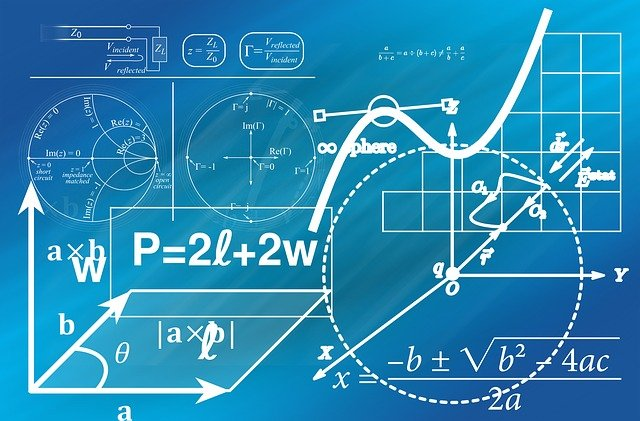Home » Blog » Maths Formulas

# Maths FormulasMaths formulas are rules written with mathematical symbols. They relate two or more quantities together with an “equal to” sign. You might want to think of them as maths made easy. In real life, maths formulas help you find the value of an unknown quantity from other known quantities.

## Examples Of Maths Formulas

Say you are building on a rectangular plot of land, and you need to fence in your property. How would you arrive at the length of fencing required for your property? Just use the maths formula for perimeter: 2 times (length + breadth)! And if you want to know what space you have within the confines of your property, use the maths formula for area: length multiplied by breadth.

Not all maths formulas are as simple as the ones stated above. Some can be complex. An example is the Harmonic Series, a sequence of numbers that keep getting smaller and tending towards infinity as you add them. Or Euler’s identity, which relates the seemingly random values of pi, e, and the square root of -1.

## No Maths Formulas, No Life

Maths formulas are part and parcel of our everyday lives. Human existence would be impossible without them. They help us make intelligent decisions by making us understand how things relate. So whether we want to fly astronauts into space or dig for treasure in the earth’s belly, they always come through for us.